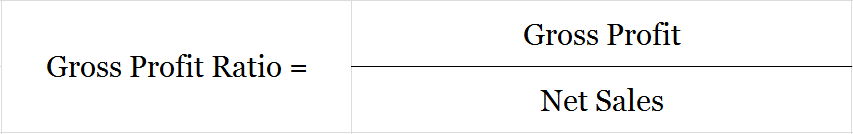# What is Gross Profit Ratio?

## Gross Profit Ratio

Also known as the Gross Profit Margin ratio, it establishes a relationship between gross profit earned and net revenue generated from operations (net sales). The gross profit ratio is a profitability ratio expressed as a percentage hence it is multiplied by 100.

Net sales consider both Cash and Credit Sales, on the other hand, gross profit is calculated as Net Sales minus COGS. The gross profit ratio helps to ascertain optimum selling prices and improve the efficiency of trading activities.

It also helps find out the lowest selling price of goods per unit to an extent that the business will not suffer a loss.

## Formula to Calculate Gross Profit RatioNote  – It is represented as a percentage so it is multiplied by 100.

Gross Profit = Net Sales – COGS

COGS = Opening Stock + Purchases + Direct Expenses* – Closing Stock

*Only used if they are specifically provided

Net Sales  = Cash Sales + Credit Sales – Sales Returns

## Example

Ques. Calculate the GP ratio from the below information

 Sales 7,00,000 Sales Returns 1,00,000 Cost of Goods Sold 3,00,000

Gross Profit Ratio = (Gross Profit/Net Sales)*100

Net Sales = Sales – Returns

7,00,000 – 1,00,000

= 6,00,000

Gross Profit = Net Sales – COGS

6,00,000 – 3,00,000

= 3,00,000

GP Ratio = (3,00,000/6,00,000)*100

= 50%

This means that for every 1 unit of net sales, the company earns 50% as gross profit. Alternatively, the company has a gross profit margin of 50%, i.e. 0.50 units of gross profit for every 1 unit of revenue generated from operations.

## High and Low Gross Profit Ratio

A business is rarely judged by its Gross Profit ratio, it is only a mild indicator of the overall profitability of the company.

High – A high ratio may indicate high net sales with a constant cost of goods sold or it may indicate a reduced COGS with constant net sales.

Low – A low ratio may indicate low net sales with a constant cost of goods sold or it may also indicate an increased COGS with stable net sales.